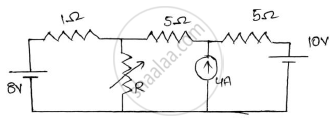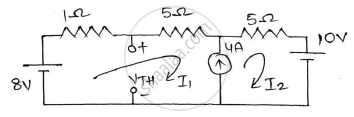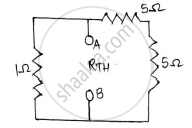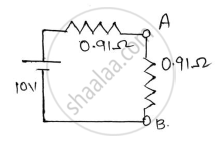# Determine the value of R for maximum power transfer and find the value of maximum transfer. - Basic Electrical and Electronics Engineering

Sum

Determine the value of R for maximum power transfer and find the value of maximum transfer.#### Solution

(1) Calculation of 𝑉𝑇𝐻
Removing the variable resistor R from the networkMesh 1 and 2 will form A loop.
Writing current equation for the loop.
𝐼2−𝐼1=4 ………………..(1)
Applying KVL to the loop,
8−𝐼1−5𝐼1−5𝐼2−10=0
−6𝐼1−5𝐼2=2 ………..(2)
From (1) and (2) we get,
𝐼1=−2𝐴 and 𝐼2=2𝐴
Writing 𝑉𝑇𝐻 equation,

8−𝐼1−𝑉𝑇𝐻=0 => 8+2−𝑉𝑇𝐻=0
𝐕𝐓𝐇=𝟏𝟎𝐕
(2) Calculation of 𝑅𝑇𝐻Replacing the voltage source by short circuits and current sources by an open circuit
𝑅𝑇𝐻 = 10Ω || 1Ω = 0.91Ω
For maximum power transfer
(3) Calculation of 𝑃𝑚𝑎𝑥

P_(max)=(V_(TH)^2)/(4R_(TH))=10^2/(4xx0.91)=27.47wConcept: Maximum Power Transfer Theorem
Is there an error in this question or solution?
2018-2019 (December) CBCGS

Share